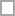# Option Quizzes Online & Trivia

A comprehensive database of option quizzes online, test your knowledge with option quiz questions. Our online option trivia quizzes can be adapted to suit your requirements for taking some of the top option quizzes.

## Option Questions and Answers

• Using the Black Scholes formula, calculate the price of a 4-month European call option on the British pound. You are given the following details: The current exchange rate is 1.3, the exercise price is 1.3. The risk free interest rate in the United States is 3% per annum whereas the risk free rate in Britain is 4% per annum. The annualized implied volatility in the exchange rate is 20%. The European call option price is:Option question from

• What are the values of u, d and p when a binomial tree is constructed to value an option on a foreign currency. The tree step size is 1 month, the domestic interest rate is 5% per annum, the foreign interest rate is 8% per annum, and the volatility is 12% per annum.Option question from

• Use the conventional binomial tree method with n=3 steps to calculate the price of a 4-month American put option on the British pound. You are given the following details: The current exchange rate is 1.3, the exercise price is 1.3. The risk free interest rate in the United States is 3% per annum whereas the risk free rate 4% per annum. The annualized implied volatility in the exchange rate is 10%. The price of the American put option is:Option question from

• What does NOMS stand for?Option question from

• What is the role of the NOMS?Option question from

• What is the role of the Crown Prosecution ServiceOption question from

• Did you enjoy school?Option question from

• Do you do well in exams?Option question from

• Do you want to continue studying in a classroom environment?Option question from